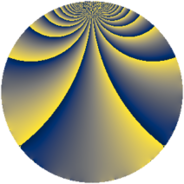Properties

 Label 448.1.rLevel $448$ Weight $1$ Character orbit 448.r Rep. character $\chi_{448}(191,\cdot)$ Character field $\Q(\zeta_{6})$ Dimension $4$ Newform subspaces $1$ Sturm bound $64$ Trace bound $0$

Related objects

Defining parameters

 Level: $$N$$ $$=$$ $$448 = 2^{6} \cdot 7$$ Weight: $$k$$ $$=$$ $$1$$ Character orbit: $$[\chi]$$ $$=$$ 448.r (of order $$6$$ and degree $$2$$) Character conductor: $$\operatorname{cond}(\chi)$$ $$=$$ $$28$$ Character field: $$\Q(\zeta_{6})$$ Newform subspaces: $$1$$ Sturm bound: $$64$$ Trace bound: $$0$$

Dimensions

The following table gives the dimensions of various subspaces of $$M_{1}(448, [\chi])$$.

Total New Old
Modular forms 36 8 28
Cusp forms 12 4 8
Eisenstein series 24 4 20

The following table gives the dimensions of subspaces with specified projective image type.

$$D_n$$ $$A_4$$ $$S_4$$ $$A_5$$
Dimension 0 4 0 0

Trace form

 $$4 q + 2 q^{5} + O(q^{10})$$ $$4 q + 2 q^{5} - 2 q^{17} + 2 q^{21} - 2 q^{33} - 2 q^{37} - 4 q^{49} - 2 q^{53} - 4 q^{57} - 2 q^{61} - 4 q^{69} + 2 q^{73} - 2 q^{77} + 2 q^{81} - 4 q^{85} + 2 q^{89} + 2 q^{93} + O(q^{100})$$

Decomposition of $$S_{1}^{\mathrm{new}}(448, [\chi])$$ into newform subspaces

Label Dim. $$A$$ Field Image CM RM Traces $q$-expansion
$a_{2}$ $a_{3}$ $a_{5}$ $a_{7}$
448.1.r.a $4$ $0.224$ $$\Q(\zeta_{12})$$ $A_{4}$ None None $$0$$ $$0$$ $$2$$ $$0$$ $$q+\zeta_{12}q^{3}+\zeta_{12}^{2}q^{5}-\zeta_{12}^{3}q^{7}-\zeta_{12}q^{11}+\cdots$$

Decomposition of $$S_{1}^{\mathrm{old}}(448, [\chi])$$ into lower level spaces

$$S_{1}^{\mathrm{old}}(448, [\chi]) \cong$$ $$S_{1}^{\mathrm{new}}(224, [\chi])$$$$^{\oplus 2}$$